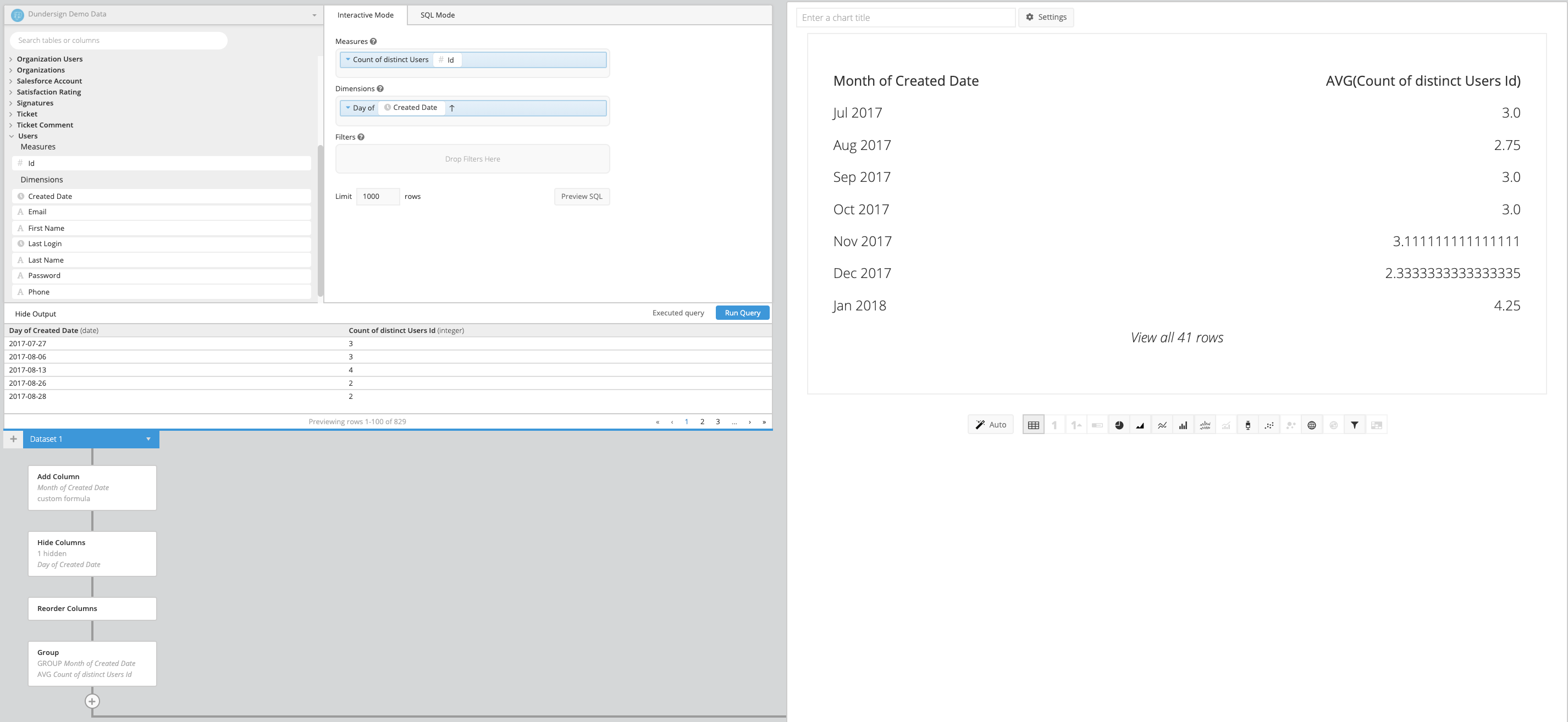# Group Step example for Data Explorer

A common metric that lots of companies are interested in knowing is the monthly average of the count of users created per day. You can calculate this using a nested SELECT statement in SQL Mode or do it in Interactive Mode in just a few steps.

For this example, we use columns from the Users table in our Dundersign Demo Data data source.

1. First, get a count of users created each day. Do this by dragging the Id column to the Measures field and using the Count of distinct aggregation. Next, drag the Created Date column to the Dimensions field and use the default Day time bucket. Click Run Query.

Your result set might look something like the image below:

Note: If you don’t need to use the full date column in your chart before calculating the average, you can instead group your Created Date column by the Month time bucket here and skip to step 6.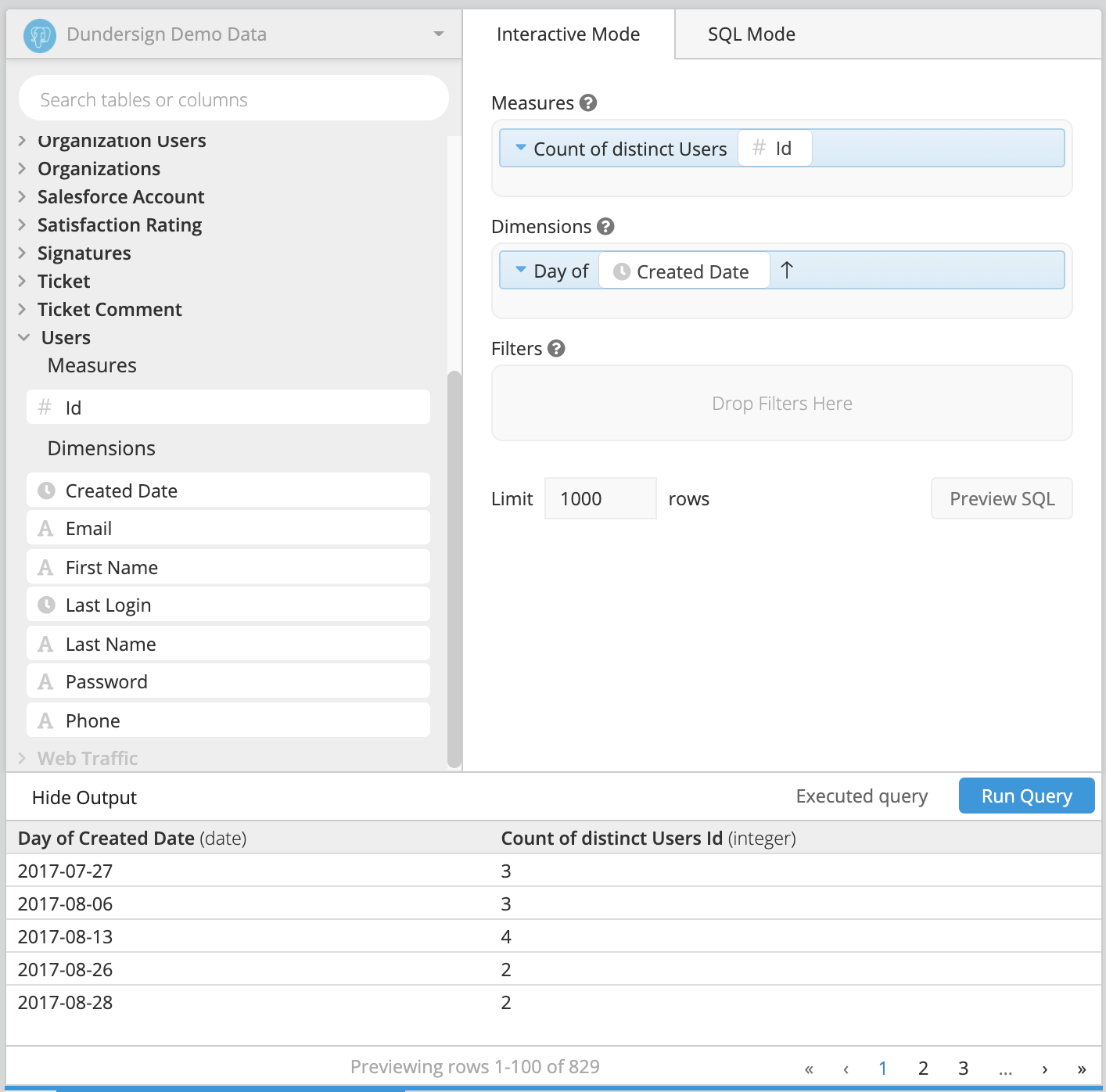2. Next, we’ll want to create a column that shows the month and year of Created Date, so we can use it later to group by it.

To do this, click + Add Transformation in the Data Pipeline and choose Add Column from the list of available options.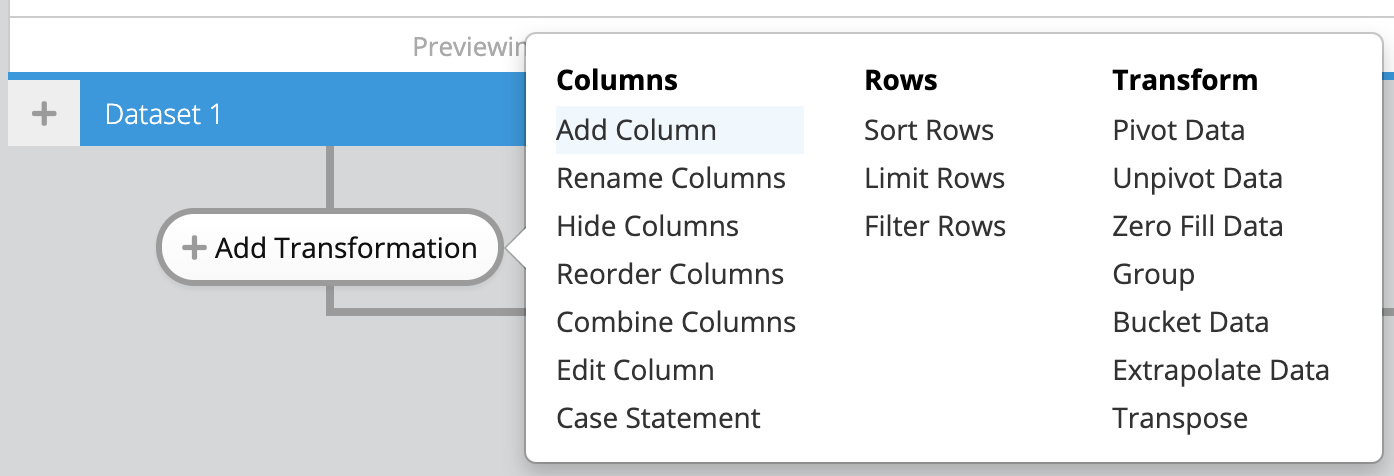3. In the Add Column step, we’ll use SQLite’s [strftime() function](https://www.sqlite.org/lang_datefunc.html “striftime() function below for the formula, which extracts only the month and year from the “Day of Created Date” column. We’ll use this column to group the average number of new users per month.

`````` strftime('%Y-%m',"Day of Created Date")
``````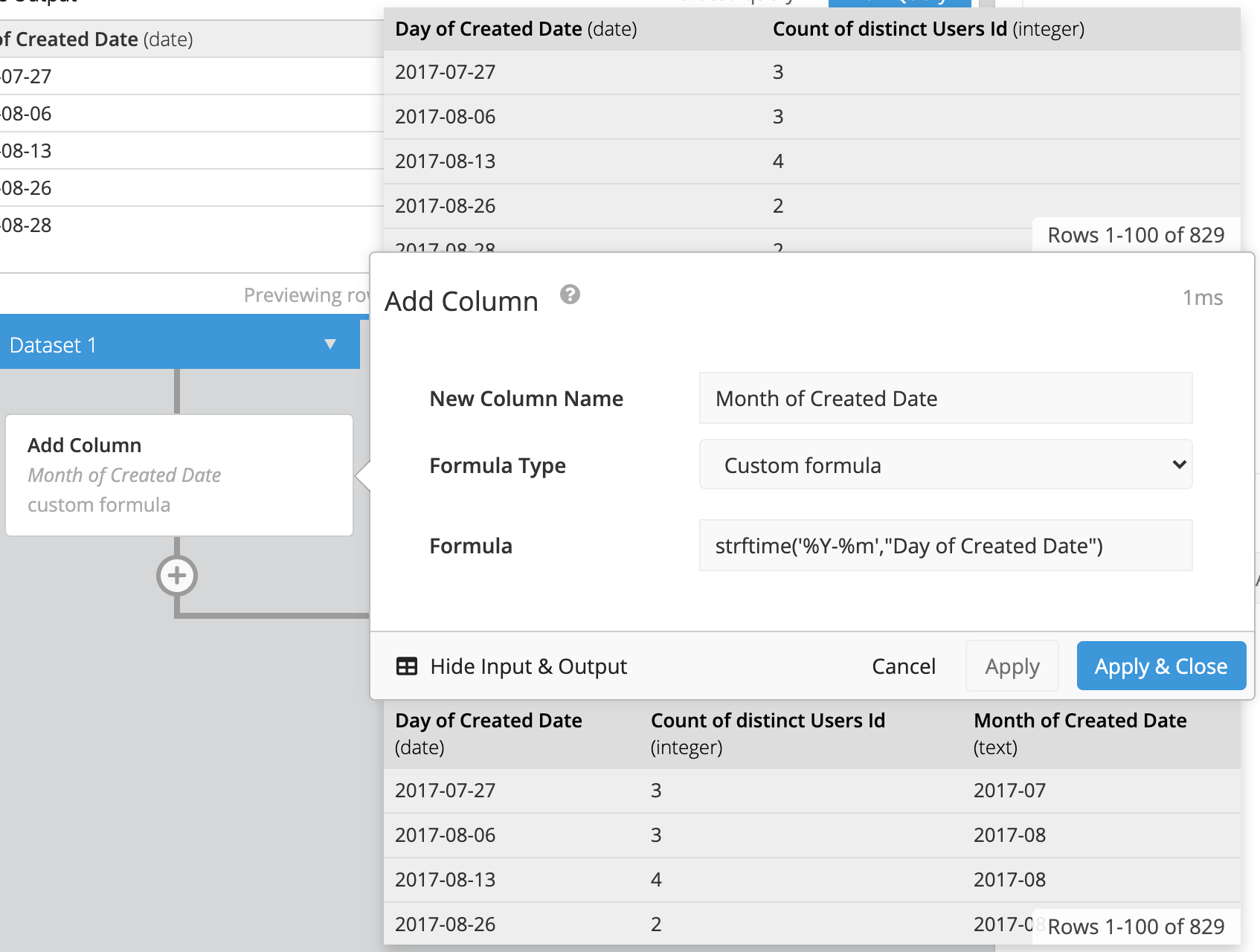4. We’ll then want to hide the “Day of Created Date” column since it is no longer needed using a Hide Columns step.
5. Then, add a Reorder Columns step to reorder the columns so that “Month of Created Date” is the first column and “Count of distinct Users Id” is the second one in the chart.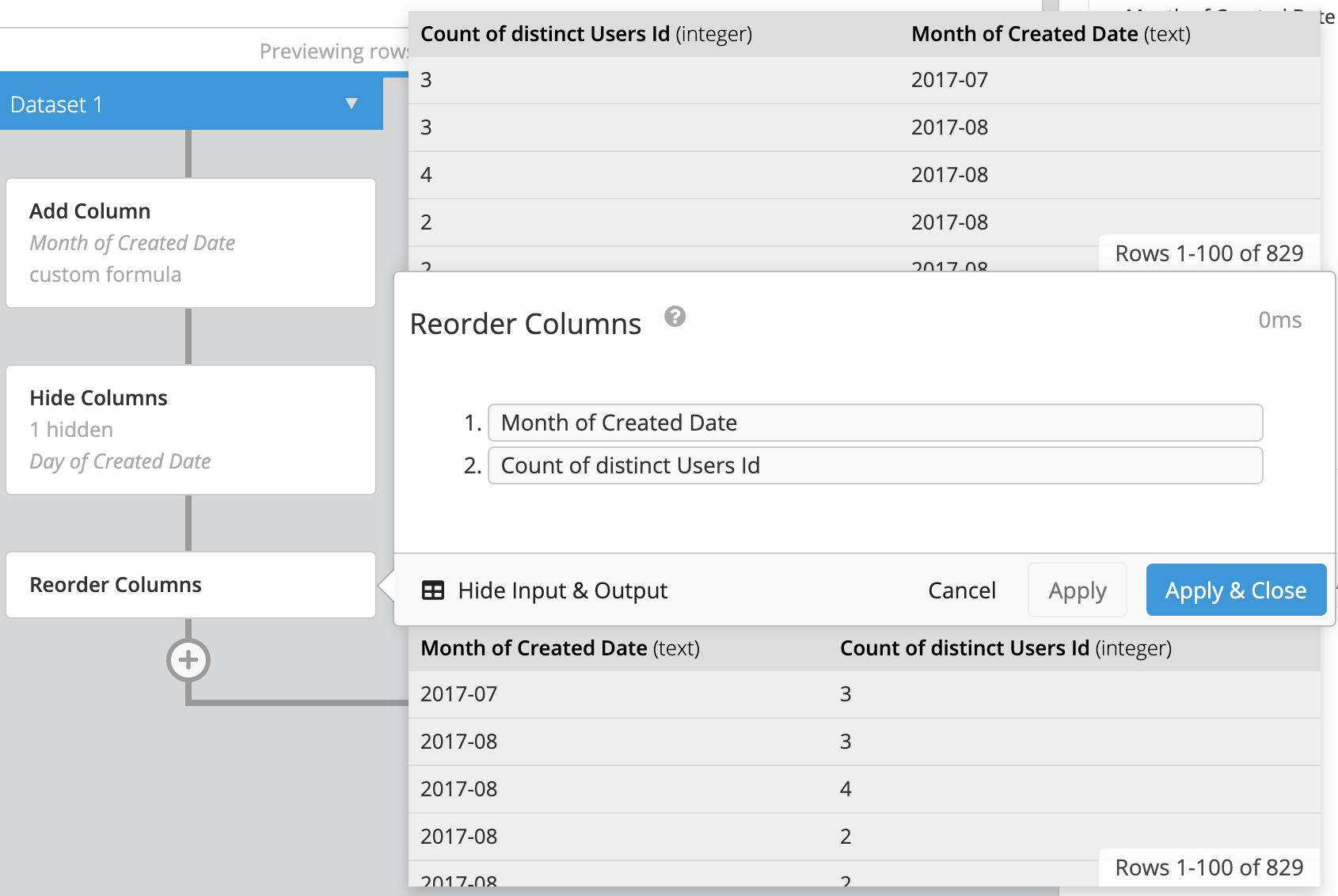6. And now for the most important part: Add a Group step in the Data Pipeline. We want to choose GROUP as the aggregation for “Month of Created Date” because we’ll use this column to group the “Count of distinct Users Id” by month. Since we want the average count of users, we’ll then choose AVG as the aggregation for the “Count of distinct Users Id” column. Click Apply & Close.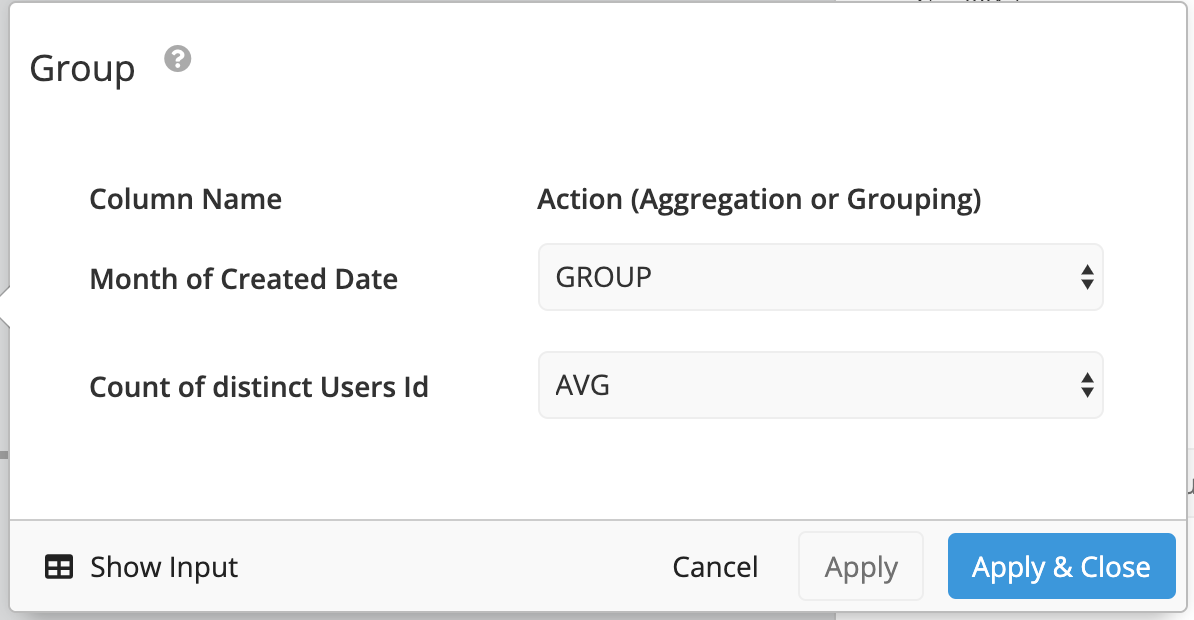This process negates the need to write a nested select statement and can be done in just a few minutes. A full preview of all of the steps needed to create this chart can be found below: